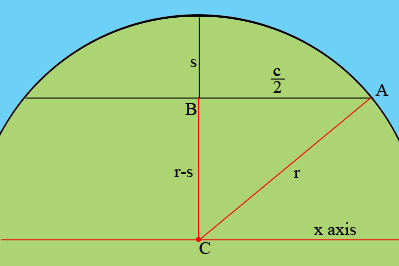SEARCH HOMEMath Central Quandaries & QueriesQuestion from Isiah, a student: So I am working on a problem with a few friends; you have an arc with the center of its chord at 0,0. We also have a known sagitta and a known radius of curvature. How do we calculate the height extending in both the positive and negative directions? Central sag: 2.48 Chord length: 9.6Hi Isiah,

I am going to change your setup slightly. I want to find the equation of the circle and since the equation of a circle is easiest

$x^2 + y^2 = r^2 \mbox{ where r is the radius,}$

when the central of the circle is at the origin I am going to move $(0, 0)$ to the centre of the circle.In my diagram $s$ is the length of the sagitta, $c$ is the length of the chord, $r$ is the unknown radius of the circle nd $C (0,0)$ is the centre of the circle.

Triangle ABC is a right triangle so a use of Pythagoras Theorem will allow you to solve for $r.$ Once you have the radius of the circle you can use the equation

$y = \sqrt{r^2 - x^2}$

to solve for $y$ for points at a distance $x$ from $C$ along the x axis. At each such point the height of the arc above the chord is $y - (r - s).$

PennyMath Central is supported by the University of Regina and the Imperial Oil Foundation.